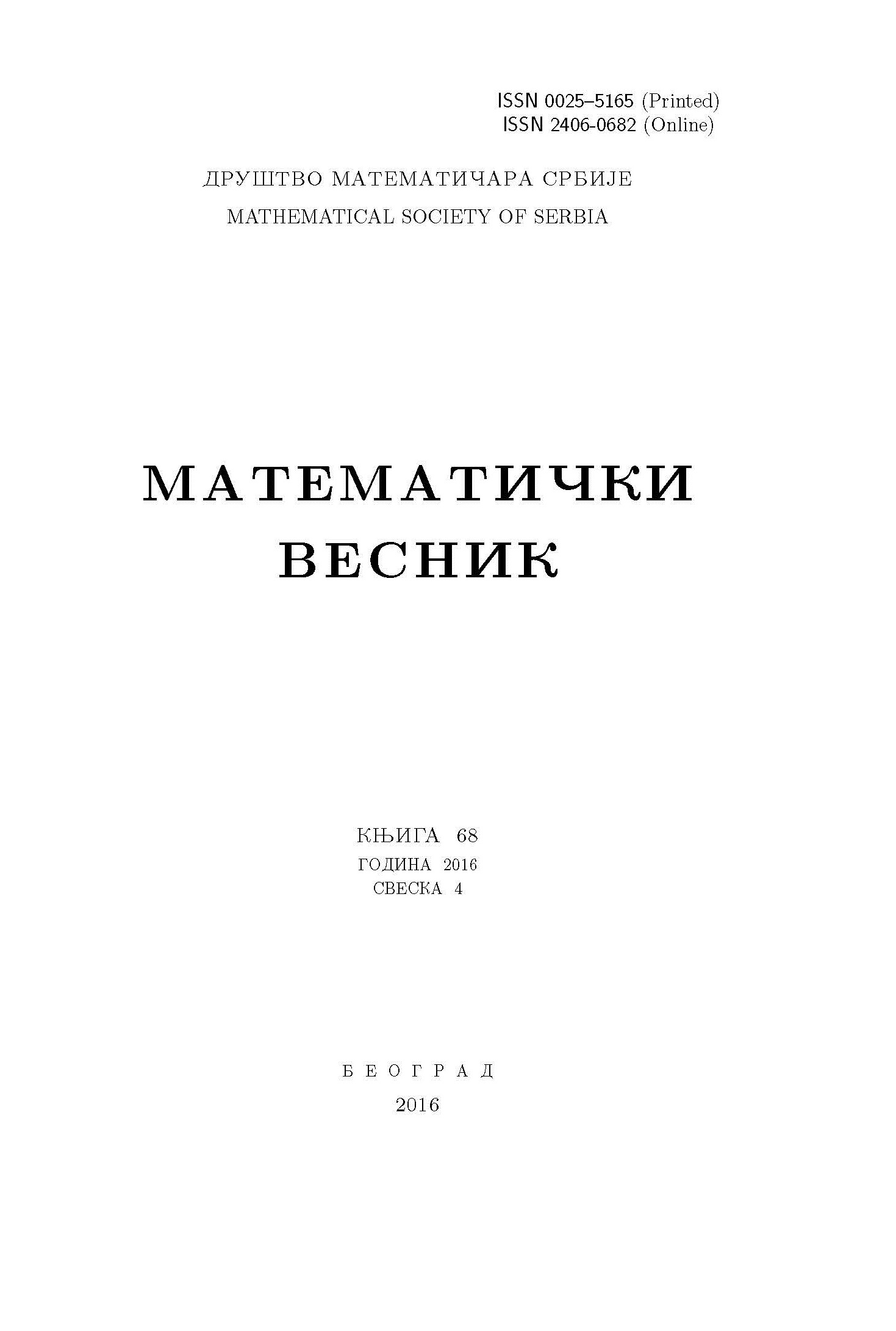﻿ Matematički Vesnik ﻿
 MATEMATIČKI VESNIK МАТЕМАТИЧКИ ВЕСНИКON ALGEBROID FUNCTIONS WITH UNIFORM SCHWARZIAN DERIVATIVE A. Fernández Arias AbstractThe question of determining under which conditions the Schwarzian derivative of an algebroid function turns out to be a uniform meromorphic function in the plane is considered. In order to do this the behaviour of the Schwarzian derivative of an algebroid function $w(z)$ around a ramification point is analyzed. It is concluded that in case of a uniform Schwarzian derivative $S_{w}(z)$, this meromorphic function presents a pole of order two at the projection of the ramification point, with a rational coefficient $\gamma_{-2}$, where $0<\gamma_{-2}<1.$ A class of analytic algebroid functions with uniform Schwarzian derivative is presented and the question arises whether it contains all analytic algebroid functions with this property.Keywords: Schwarzian derivative; algebroid function; Möbius transformation; ramification point. MSC: 30B40, 30F99 Pages:  1$-$14 Volume  74 ,  Issue  1 ,  2022

﻿